### Dyne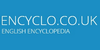A unit of force equal to the force required to accelerate a 1-g mass 1 cm per second per second. Compare with Newton.

### dyne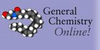(dyn) The unit of force in the obsolete cgs system of units. A dyne is the force required to accelerate a 1 g mass by 1 cm/s per second.
Found on http://antoine.frostburg.edu/chem/senese/101/glossary/d.shtml

### DyneThe dyne (symbol `dyn`, from Greek δύναμις (dynamis) meaning power, force) is a unit of force specified in the centimetre-gram-second (CGS) system of units, a predecessor of the modern SI. One dyne is equal to 10 µN (micronewtons), or to 10 nsn (nanosthenes) in the old metre-tonne-second system of units. Equivalently, the dyne is defined.....
Found on http://en.wikipedia.org/wiki/Dyne

### Dyne• (n.) The unit of force, in the C. G. S. (Centimeter Gram Second) system of physical units; that is, the force which, acting on a gram for a second, generates a velocity of a centimeter per second.
Found on http://thinkexist.com/dictionary/meaning/dyne/

### dyne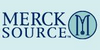(dīn) a unit of force, being the amount that when acting continuously upon a mass of 1 g will impart to it an acceleration of 1 cm per second per second. It is equal to 10−5newton.
Found on http://www.encyclo.co.uk/local/21001

### dynenoun a unit of force equal to the force that imparts an acceleration of 1 cm/sec/sec to a mass of 1 gram
Found on https://www.encyclo.co.uk/local/20974

### DyneDyne noun [ Formed from Greek ... power. See Dynamic .] (Physics) The unit of force, in the C. G. S. (Centimeter Gram Second) system of physical units; that is, the force which, acting on a gram for a second, generates a velocity of a centimeter per second.
Found on http://www.encyclo.co.uk/webster/D/135

### Dyne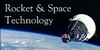A unit of force equal to the force required to accelerate a 1 g mass 1 cm per square second.
Found on http://www.braeunig.us/space/glossary.htm

### Dyne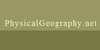A unit of force that creates an acceleration on a mass of 1 gram equal to 1 centimeter per second. 105 dynes equals one newton.
Found on http://www.physicalgeography.net/physgeoglos/d.html

### dyne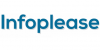dyne (dīn) , unit of force in the cgs system of units, which is based on the metric system; an acceleration of 1 centimeter per second per second is produced when a force of 1 dyne is exerted on a mass of 1 gram. In terms of the newton, the force unit in the mks system, 1 dyne equals 0.00001 n...

### dyneThe absolute centimeter-gram-second unit of force; that force that will impart to a free mass of one gram an acceleration of one centimeter per second per second.
Found on http://www.daviddarling.info/encyclopedia/D/AE_dyne.html

### Dyne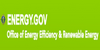The absolute centimeter-gram-second unit of force; that force that will impart to a free mass of one gram an acceleration of one centimeter per second per second.
Found on https://energy.gov/eere/energybasics/articles/glossary-energy-related-terms

### Dyne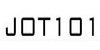The absolute unit of force
Found on http://jot101.com/2015/05/a-z-of-science-fiction-words/

### Dyne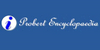The dyne is the unit of force. One dyne is that force which, acting on a mass of one gram, imparts to it an acceleration of one centimetre per second per second.
Found on http://www.probertencyclopaedia.com/browse/GD.HTM

### DyneThe unit of force in the cgs (centimeter grams second) system of units. A force of 1 dyne acting on a mass of 1g imparts an acceleration of 1cms-2.ttle='Force';xiunt='dyne';yiunt='N';mconv=1e5;cconv=0.0; See also: CGS Units, Force.
Found on http://www.diracdelta.co.uk/science/source/d/y/dyne/source.html

### Dyne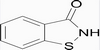The unit of force in the obsolete cgs system of units. A dyne is the force required to accelerate a
Found on http://www.superglossary.com/Glossary/Science/Chemistry/

### dyne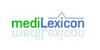Type: Term Pronunciation: dīn Definitions: 1. The unit of force in the CGS system, replaced in the SI system by the newton (1 N = 105 dynes), that gives a body of 1 g mass an acceleration of 1 cm/sec2; expressed as F (dynes) = m (grams) ׀ a (cm/sec2).
Found on http://www.medilexicon.com/medicaldictionary.php?t=27160

### dyneunit of force in the centimetre-gram-second system of physical units, equal to the force that would give a free mass of one gram an acceleration of ...
Found on http://www.britannica.com/eb/a-z/d/88

### dyne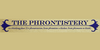unit of force to accelerate 1 gram to 1 cm per second per second
Found on http://phrontistery.info/d.html

### dyne[n] - a unit of force equal to the force that imparts an acceleration of 1 cm/sec/sec to a mass of 1 gram
Found on http://www.webdictionary.co.uk/definition.php?query=dyne
No exact match found